VLOOKUP is one of the popular functions in Excel.

It is very helpful to many Excel users who want to look and retrieve information from a table (or dataset).

As important as it is, VLOOKUP isn’t flawless. It possesses some limitations.

If you know these VLOOKUP limitations, and the techniques you can employ to get around them, then you can solve a lot of problems in Excel.

Without so much ado, below are some of the limitations of VLOOKUP function and where to find their solutions.

## VLOOKUP cannot look left

This seems to be the greatest VLOOKUP limitation for most Excel users.

The function is designed in a way that it can only retrieve data from the columns at the right handside, once it finds the lookup value.

Check the data below for example, the VLOOKUP function cannot look-up a date in the 5th (or last) column and retrieve the product that was sold on that date.

This is because VLOOKUP is only designed to look to the right direction after the lookup column.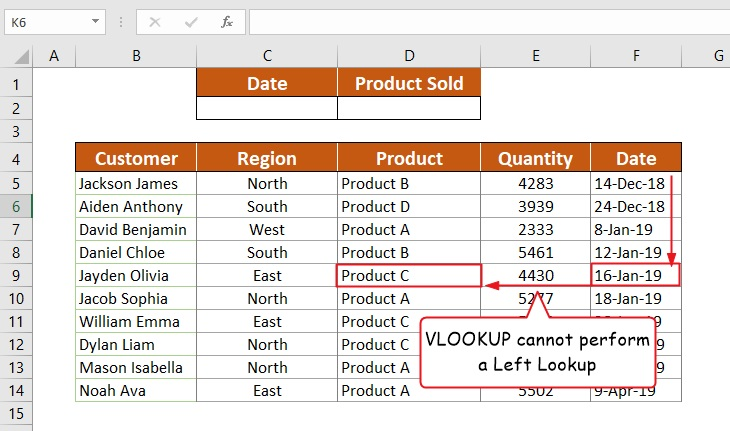This is a limitation because it limits your choice of presenting data the way you want. If the column from which you wish to retrieve data is located to the left of the lookup column, then you’ll have to change your order of columns in your data table by rearranging them to suit VLOOKUP needs.

## A suggested solution to this limitation

The most popular way to skirt this VLOOKUP limitation is to switch to its rival functions – INDEX-MATCH.

The =INDEX() and =MATCH() functions, when used together, can overcome this VLOOKUP limitation. You can use them together to perform a lookup to any direction in the lookup table (something VLOOKUP can’t afford).

Let’s take the above example for instance, with the INDEX-MATCH, you can look-up a date and retrieve corresponding data in any column to the left, say product. See it in the screenshot below: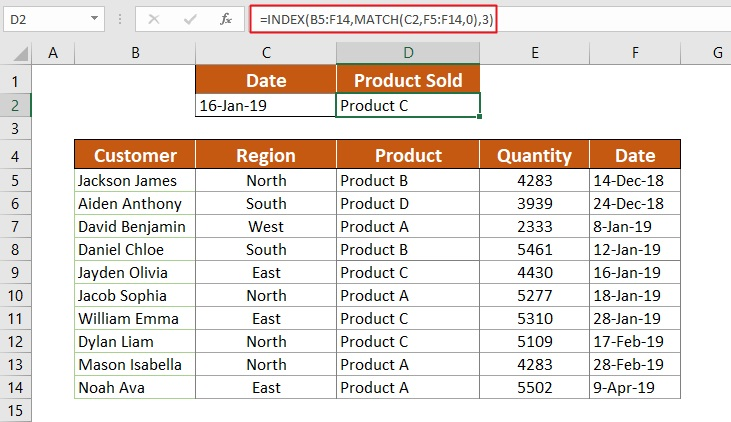Useful link: VLOOKUP to the left using INDEX-MATCH.

## VLOOKUP cannot lookup 2 columns

Normally, VLOOKUP will look through the first column of your specified range to find your search term. What if you want to fetch data based on two columns? This is when you’ll have a problem. Consider the example below:Let’s say you want to look for a customer named David and fetch the date on which you sold to this customer.

Looking at the table above, David appears two times.

If you use VLOOKUP to perform this task, it’ll use the first match and return 8-Jan-19 as a result.

Technically, this is correct, and if you are looking for David from the West region, it is correct too. However, if you are looking for David from the North region, then you should be concern over the result.

If only VLOOKUP could work with multiple criteria and fetch the date based on the two columns (David who’s also from the North). This is another shortcoming of the almighty VLOOKUP function. VLOOKUP 2 criteria are not possible

## A suggested solution to this limitation

To get around this restriction, you’ll have to use the following technique. I learned this from Samit Bansal of trumpexcel.com.

### Using a helper column

What this technique teaches is very simple. You want VLOOKUP to look into two separate columns (which it cannot). Why not combine those two columns into a single column, then look into the combined column instead?

The combined column will then be a helper column (i.e. for the purpose of the lookup only).

See below what I am talking about: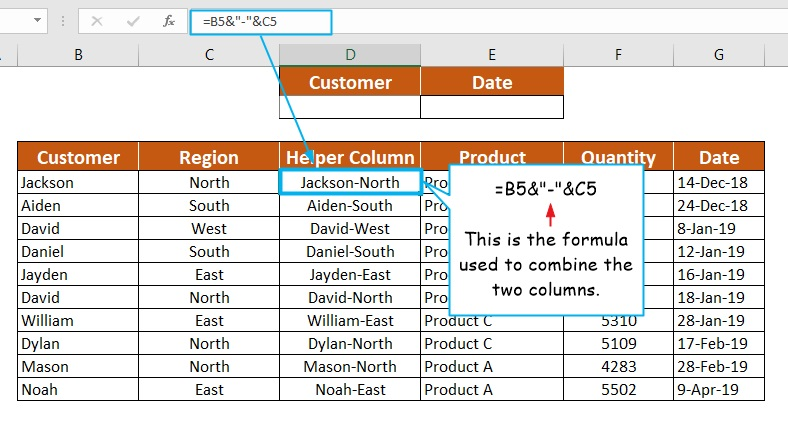Now that you have a helper column, you can use VLOOKUP to look through it.

However, when specifying the lookup value, you should use a hyphen (-) to separate the two values because the helper column used it.

See screenshot: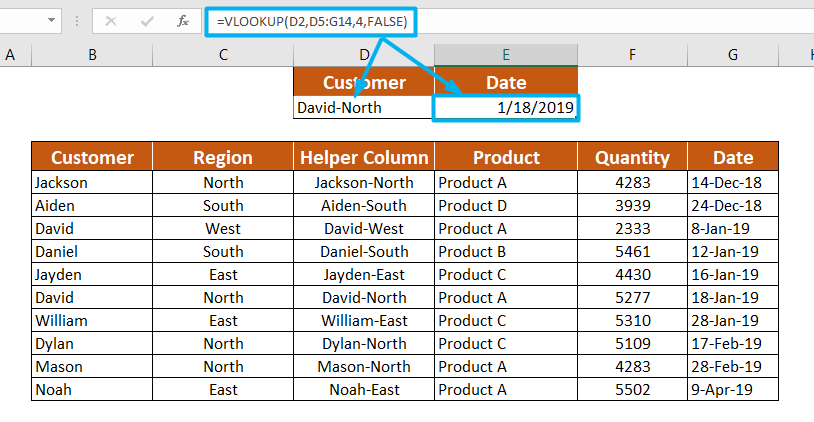## VLOOKUP cannot look at both directions

Some will say two-way lookup. Others will say double lookup. Whatever you call it, it is simply a formula that looks in both vertical and horizontal directions simultaneously. Such a formula carries along with both the powers of vertical and horizontal lookup.

If only these two functions (VLOOKUP and HLOOKUP) could work together, it could have been great for this task. But no, both functions are crippled when it comes to double lookup. So, let’s just say this another limitation of the VLOOKUP function.

## A suggested solution to this limitation

Whenever VLOOKUP fails, the INDEX-MATCH functions almost always rules. With these two functions, you can look in both directions.

Let’s take the table below for an example. Imagine you want to write a formula that will return a student’s marks based on the name and the type of test you specified.

VLOOKUP can look through the name column and return a student’s marks based on the column number you specified. However, the index number can look through the name column as well, but at the same time the heading row (first row) to retrieve a student’s marks.

See screenshot:Looking in the above example, you notice that the formula performs a two-way lookup using the INDEX and MATCH functions. It uses the MATCH function twice as arguments for the INDEX function to get the row number and column number respectively.

To understand this more, let me fine-tune the worksheet for you. See below: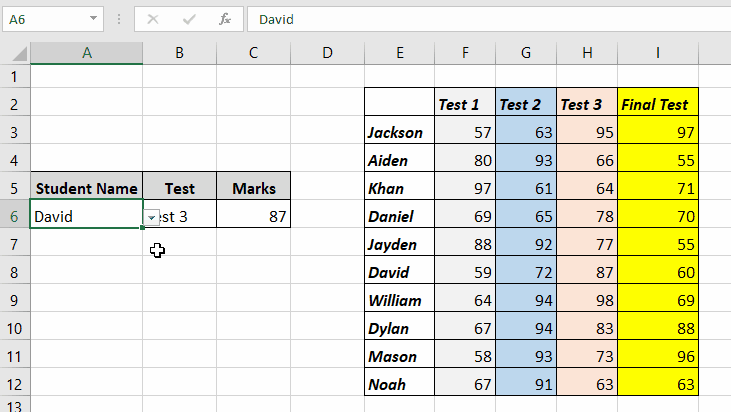However, for a more detailed explanation on how to perform a double lookup using the INDEX-MATCH functions visit this article: How to do a double lookup in Excel.

Below is the formula used to retrieve the marks in the above worksheet:

=INDEX(E2:I12,MATCH(A6,E2:E12,0),MATCH(B6,E2:I2,0))

As seen above, to retrieve someone’s marks, the spreadsheet user just need to enter a student’s name as well as the test, whether test 1, 2, 3 or final test.

The formula used in this worksheet isn’t any different form the previous one. All I did was refer to cell A6 and B6 whose values are used as the lookup values.